# The maximal order of an element in the symmetric group

Let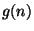be the maximal order of a permutation of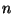objects,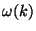be the number of distinct prime divisors of the integer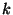and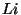be the integral logarithm.

Then RH is equivalent to each of the following statements :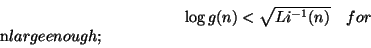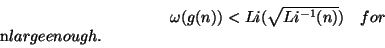This is due to Massias, Nicolas and Robin [ MR 89i:11108].

Back to the main index for The Riemann Hypothesis.